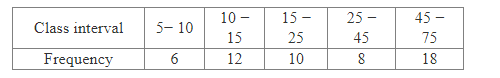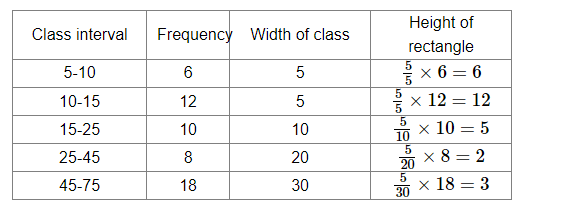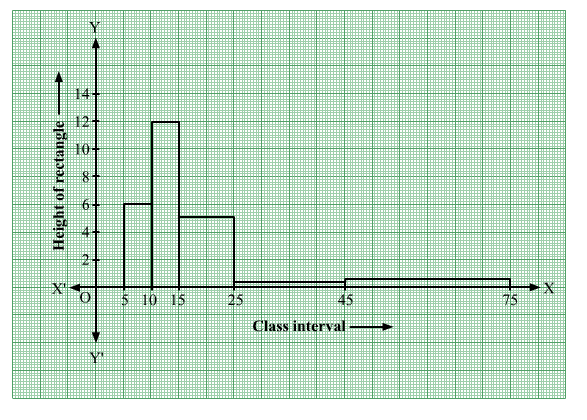# Draw a histogram to represent the following information:Question:

Draw a histogram to represent the following information:Solution:

Here the class intervals are of unequal size. So, we calculate the adjusted frequency using the formula,

Adjusted freq $=\frac{\min \text { class size }}{\text { class size of this class }} \times$ freq

Class sizes are

$10-5=5$

$15-10=5$

$25-15=10$

$45-25=20$

$75-45=30$

Minimum class size = 5